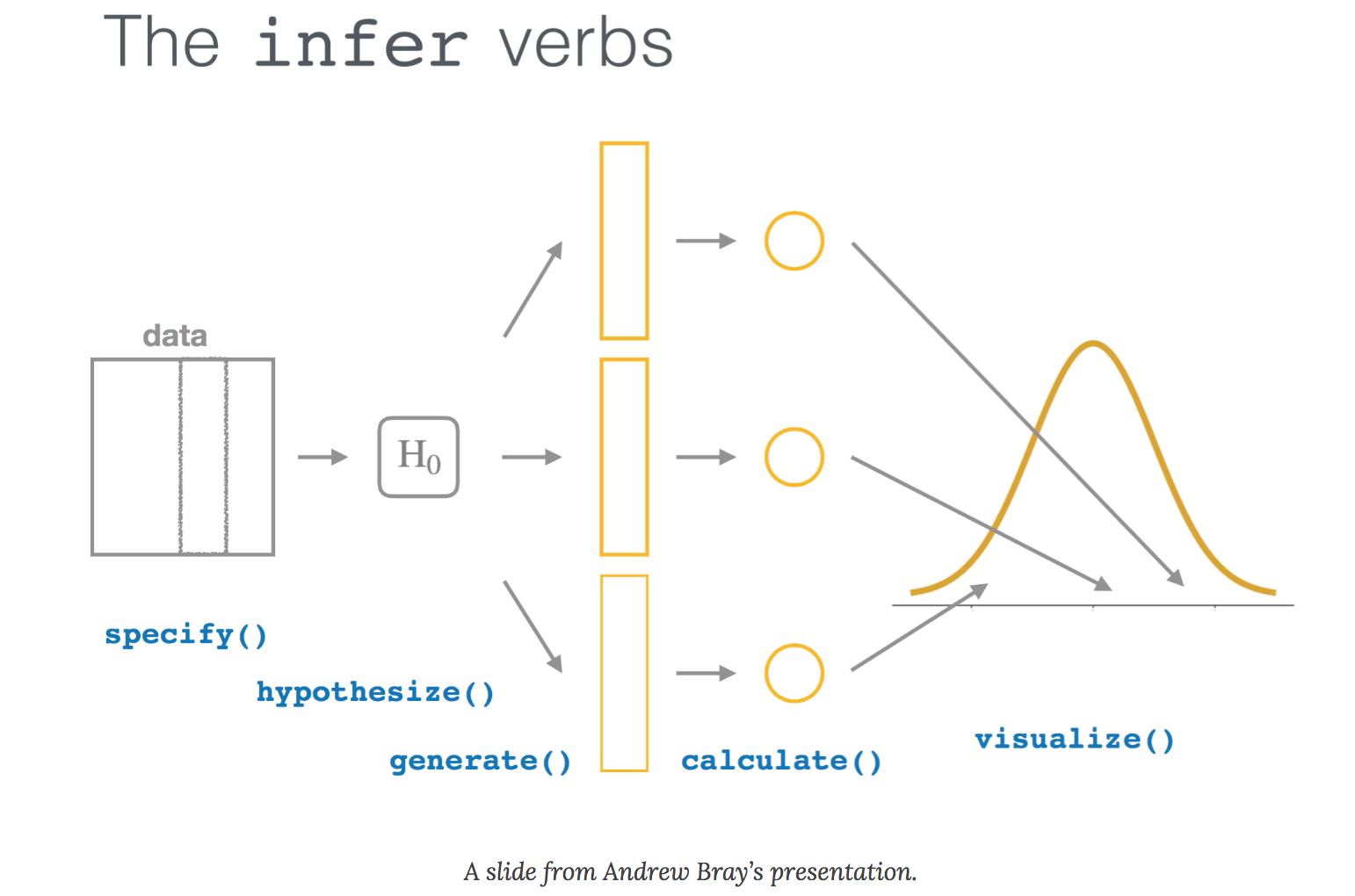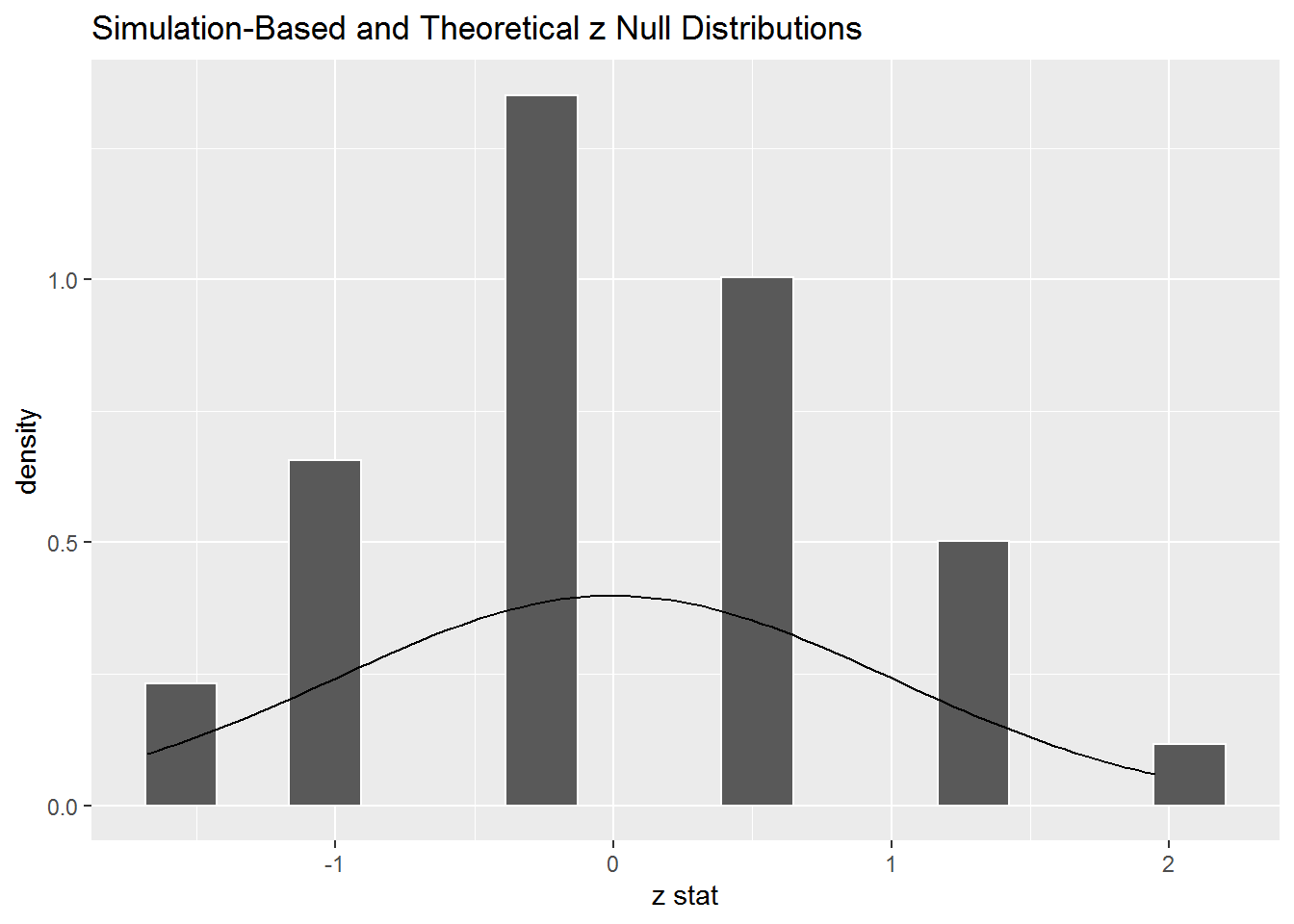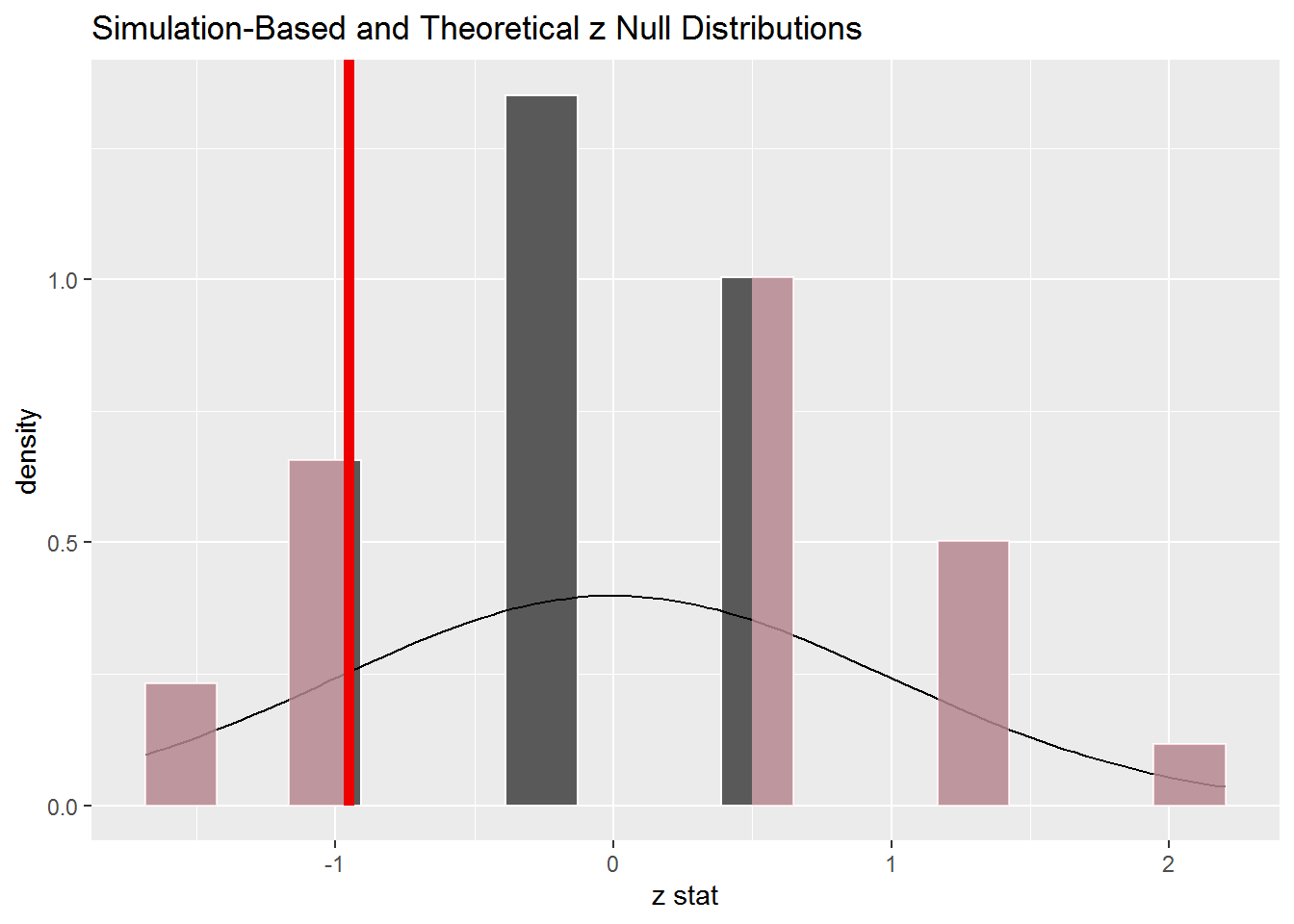# infer

· by Jo Hardin · Read in about 4 min · (804 words) ·

Although an agreed upon definition of data science is hard to come by, there is clear consensus that statistics plays a key role in the foundational knowledge of anyone working with data. One important aspect of statistics is understanding of the inferential process that allows claims to be made about a population from a dataset. Most Introductory Statistics courses and textbooks spend substantial time presenting statistical inference as a way to generate p-values and make claims (or not) about a research hypothesis. Sometimes the process of hypothesis testing is taught as a series of different test procedures (depending on the data structure and research hypothesis), and sometimes it is taught in a uniform framework. The infer package works to help students understand the creation of a sampling distribution under some model, likely the null model.

Among countless applications of statistical inference in the natural sciences and social sciences, one place that inference plays an important role in the technology field is in A/B testing. Teaching statistical inference (particularly when using the tidy pipeline provided by infer) will help students grok sampling distributions, inference, and p-values. The deep understanding will benefit students when they move from the two-sample setting to more complex models like multivariate linear or generalized linear models.

### Tidy statistical inference

Many statistics educators may have recognized the value in teaching the tidyverse and providing students a fluency with data wrangling. However, they may also have felt a disconnect between the computational tools for tidy data wrangling and the (often) central inferential ideas being taught in an introductory statistics course. That is, the majority of R inferential tests (e.g., t.test, chisq.test, etc.) do not play nicely with the tidyverse.

To connect the the tidyverse with the central inferential ideas from introductory statistics, Andrew Bray and Chester Ismay have built the R package infer. Along with creating syntax that flows seamlessly through a tidy pipeline, the brilliance of the infer package is that it breaks hypothesis testing down into its tidy components! That is, virtually all hypothesis testing (and certainly that which is taught in Introductory Statistics) can be broken town into the following infer verbs:

• specify() the variables of interest
• hypothesize() about a null claim (or don’t if you plan to create an interval)
• generate() observations from the null hypothesis or from the data set (e.g., using bootstrapping) if there is no null hypothesis
• calculate() a test statistic
• visualize() the test statistics from the null distribution as compared with the observed test statistic### Using infer

The example below is from one of the infer vignettes and walks the student through a two sample difference in proportions test. Note that the structure of the R code forces the student to think carefully about each step in the process.

library(infer)
library(dplyr)
mtcars <- mtcars %>%
mutate(vs = factor(vs),
am = factor(am))
# For reproducibility
set.seed(4747)

cars2props <- mtcars %>%
specify(am ~ vs, success = "1") %>% # alt: response = am, explanatory = vs
hypothesize(null = "independence") %>%
generate(reps = 100, type = "permute") %>%
calculate(stat = "z", order = c("0", "1")) %>%
visualize(method = "both")
cars2propsThe p-value calculation can be done with the get_p_value function. Or alternatively, the observed statistic can be calculated using the same pipeline steps (no hypothesize or generate!) and can be visualized on top of the sampling distribution created above.

obs_stat <- mtcars %>%
specify(am ~ vs, success = "1") %>%
calculate(stat = "z", order = c("0", "1"))
obs_stat
## # A tibble: 1 x 1
##     stat
##    <dbl>
## 1 -0.952

mtcars %>%
specify(am ~ vs, success = "1") %>% # alt: response = am, explanatory = vs
hypothesize(null = "independence") %>%
generate(reps = 100, type = "permute") %>%
calculate(stat = "z", order = c("0", "1")) %>%
get_p_value(obs_stat, direction = "both")
## # A tibble: 1 x 1
##   p_value
##     <dbl>
## 1     0.5

cars2props + shade_p_value(obs_stat, direction = "both")